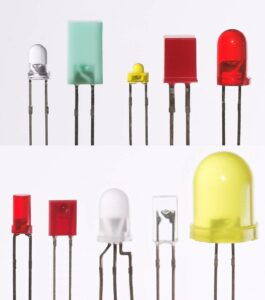# How to Calculate Resistor Value for LED Lighting

Determining the resistor value for lighting LEDs is simple and straightforward, but we must take into consideration the color of the LED as well as the wattage rating of the required resistor, and the number of LEDs in the circuit. We hope reading How to Calculate Resistor Value for LED Lighting gives you what you would need for your project.

LEDs are becoming more and more popular for a variety of lighting projects and needs. This is due to the excellent power efficiency and extended lifetimes of LEDs over incandescent lamps. Also, as the technology improves and production increases, the cost continues to be reduced.

## Follow these steps to calculate resistor value for LED Lighting by 12V, DC:

1. Determine the voltage and current needed for your LED.
2. We'll use the following formula to determine the resistor value: Resistor = (Battery Voltage – LED voltage) / desired LED current.
3. For a typical white LED that requires 10mA, powered by 12V the values are: (12-3.4)/.010=860 ohms.
4. To use several LEDs in parallel, sum the current values. From the example above, if we use 5 white LEDs the current requirement is 10mA x 5 = 50mA. So (12-3.4)/.050=172 ohms.## Calculating Resistor value for LED Lighting

######8x8x8 RGB LED Cube by GPL3+

LED is an acronym for Light Emitting Diode. This means that an LED has a specific polarity that must be applied to make it produce light. Failure to observe this polarity requirement could cause catastrophic damage to the LED. This is because an LED has a relatively low value of reverse polarity voltage that is allowed (normally about 5 volts). Since an LED is essentially a diode, it has a maximum current value that cannot be exceeded for any period of time.

## LEDs Applications

With this in mind, we will explore the requirements for the limiting resistor that must be used in an LED circuit. Since LEDs are available in various colors, the required resistance value will vary depending on the color of the LED. This is because the color of the LED is determined by the materials used to make it and these various materials have different voltage characteristics. The forward voltage value is the voltage required to cause the LED to light. Typical Red, Green, Orange, and Yellow LEDs have a forward voltage of approximately 2.0 volts; but White and Blue LEDs have a forward voltage value of 3.4 volts. Because of this variation, the value of resistor value will vary depending on what the LED color is.

The procedure is to choose a resistor value that will produce the correct amount of current to flow in the LED-based on this forward voltage value and the value of the Power supply that is powering the circuit.

Since automotive applications are one of the most popular uses for LEDs, I will go through an example of an LED lighting project that uses 12 volts as the power source. The formula required is ohm’s law which states that the Resistance is equal to the voltage divided by the Current. The important feature to note here is that the voltage value used in the calculation. The difference between the power supply (battery) voltage and the LED’s forward voltage value. This is because we want the resistor to “drop” the voltage from the power source down to the forward voltage value of the LED.

## The formula:

### Resistor = (Battery Voltage – LED voltage) / desired LED current.

So assuming a 12-volt power source and a white LED with the desired current of 10 mA; The formula becomes Resistor = (12-3.4)/.010 which is 860 ohms. Since this is not a standard value I would use an 820-ohm resistor. We also need to determine the power rating (watts) of the required resistor. This is calculated by multiplying the voltage value dropped across the resistor by the current value flowing in it. For our example above, (12-3.4) X .010 = 0.086 so we can safely use a ¼ watt resistor in this application since we should use the next highest standard wattage rating.

If more than one LED is required, multiple LEDs (of the same color) may be connected in parallel. This will maintain the same voltage requirement but the current value will increase in direct proportion to the number of LEDs. The wattage rating of the resistor may also increase. An as an example we will assume the same white LED but we will connect 5 LEDs in parallel. Therefore, the current value required will be 10 mA multiplied by 5 (.010 X 5 =.050). Using this in our formula ; (12-3.4)/.050= 172 ohms. Use the standard value of 180 ohms. The wattage rating will now be higher (12-3.4) X .050 = .43 so we need to use at least a ½ watt resistor in this case.## Conclusion

The two examples will be repeated for Red LEDs. For a single Red LED: (12-2.0)/.010= 1000 ohms which is 1K ohms and wattage rating is (12-2.0) X (.010) = .100 so ¼ watt is sufficient. For 5 Red LEDs in parallel: (12-2.0)/.05= 200 ohms which is a standard value and wattage rating is (12-2.0) X .050 = .5 so I would use a 1-watt resistor to give us some tolerance to compensate for variations in power supply voltage etc.

As we can see, determining the resistor value for lighting LEDs is simple and straightforward, but we must take into consideration the color of the LED as well as the wattage rating of the required resistor and the number of LEDs in the circuit. You can visit our store for a varied selection of LEDs and resistors.

Comments must be approved before appearing